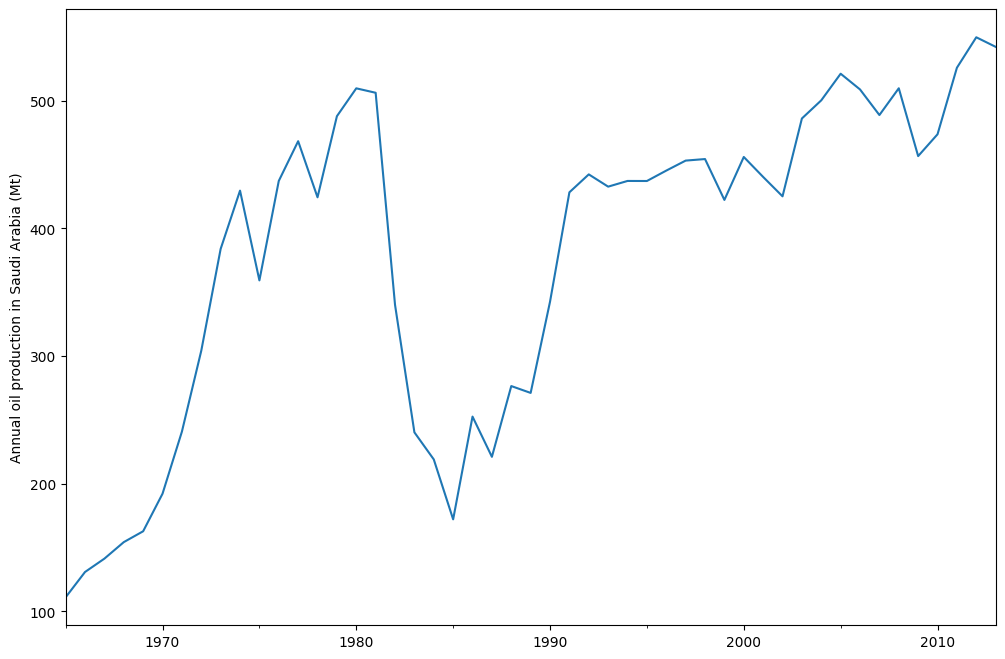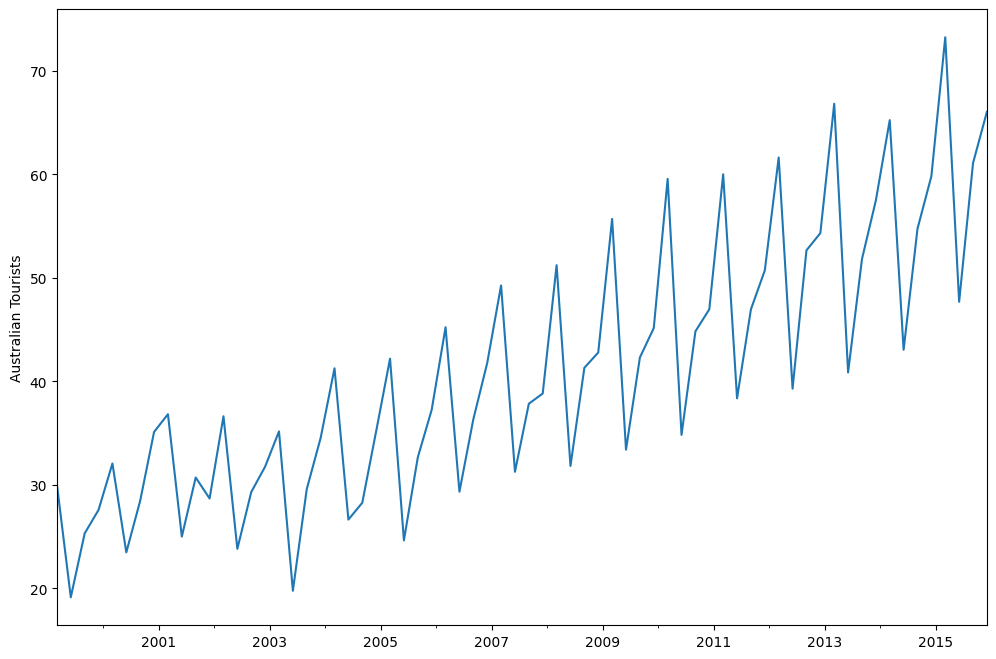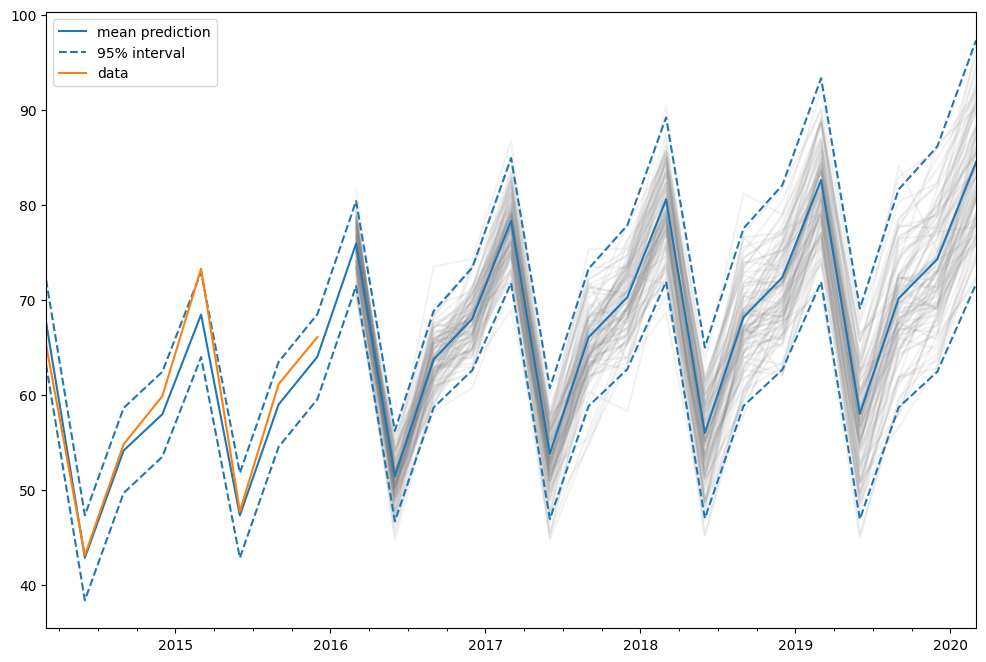# ETS models¶

The ETS models are a family of time series models with an underlying state space model consisting of a level component, a trend component (T), a seasonal component (S), and an error term (E).

This notebook shows how they can be used with statsmodels. For a more thorough treatment we refer to , chapter 8 (free online resource), on which the implementation in statsmodels and the examples used in this notebook are based.

statsmodels implements all combinations of: - additive and multiplicative error model - additive and multiplicative trend, possibly dampened - additive and multiplicative seasonality

However, not all of these methods are stable. Refer to  and references therein for more info about model stability.

 Hyndman, Rob J., and Athanasopoulos, George. Forecasting: principles and practice, 3rd edition, OTexts, 2021. https://otexts.com/fpp3/expsmooth.html

:

import numpy as np
import matplotlib.pyplot as plt
import pandas as pd

%matplotlib inline
from statsmodels.tsa.exponential_smoothing.ets import ETSModel

:

plt.rcParams["figure.figsize"] = (12, 8)


## Simple exponential smoothing¶

The simplest of the ETS models is also known as simple exponential smoothing. In ETS terms, it corresponds to the (A, N, N) model, that is, a model with additive errors, no trend, and no seasonality. The state space formulation of Holt’s method is:

\begin{align} y_{t} &= y_{t-1} + e_t\\ l_{t} &= l_{t-1} + \alpha e_t\\ \end{align}

This state space formulation can be turned into a different formulation, a forecast and a smoothing equation (as can be done with all ETS models):

\begin{align} \hat{y}_{t|t-1} &= l_{t-1}\\ l_{t} &= \alpha y_{t-1} + (1 - \alpha) l_{t-1} \end{align}

Here, $$\hat{y}_{t|t-1}$$ is the forecast/expectation of $$y_t$$ given the information of the previous step. In the simple exponential smoothing model, the forecast corresponds to the previous level. The second equation (smoothing equation) calculates the next level as weighted average of the previous level and the previous observation.

:

oildata = [
111.0091,
130.8284,
141.2871,
154.2278,
162.7409,
192.1665,
240.7997,
304.2174,
384.0046,
429.6622,
359.3169,
437.2519,
468.4008,
424.4353,
487.9794,
509.8284,
506.3473,
340.1842,
240.2589,
219.0328,
172.0747,
252.5901,
221.0711,
276.5188,
271.1480,
342.6186,
428.3558,
442.3946,
432.7851,
437.2497,
437.2092,
445.3641,
453.1950,
454.4096,
422.3789,
456.0371,
440.3866,
425.1944,
486.2052,
500.4291,
521.2759,
508.9476,
488.8889,
509.8706,
456.7229,
473.8166,
525.9509,
549.8338,
542.3405,
]
oil = pd.Series(oildata, index=pd.date_range("1965", "2013", freq="AS"))
oil.plot()
plt.ylabel("Annual oil production in Saudi Arabia (Mt)")

:

Text(0, 0.5, 'Annual oil production in Saudi Arabia (Mt)')The plot above shows annual oil production in Saudi Arabia in million tonnes. The data are taken from the R package fpp2 (companion package to prior version ). Below you can see how to fit a simple exponential smoothing model using statsmodels’s ETS implementation to this data. Additionally, the fit using forecast in R is shown as comparison.

:

model = ETSModel(oil)
fit = model.fit(maxiter=10000)
oil.plot(label="data")
fit.fittedvalues.plot(label="statsmodels fit")
plt.ylabel("Annual oil production in Saudi Arabia (Mt)")

# obtained from R
params_R = [0.99989969, 0.11888177503085334, 0.80000197, 36.46466837, 34.72584983]
yhat = model.smooth(params_R).fittedvalues
yhat.plot(label="R fit", linestyle="--")

plt.legend()

RUNNING THE L-BFGS-B CODE

* * *

Machine precision = 2.220D-16
N =            2     M =           10

At X0         0 variables are exactly at the bounds

At iterate    0    f=  6.27365D+00    |proj g|=  8.99900D-01

At iterate    1    f=  5.31675D+00    |proj g|=  6.49880D-04

At iterate    2    f=  5.30939D+00    |proj g|=  5.55467D-04

At iterate    3    f=  5.29115D+00    |proj g|=  5.87086D-05

At iterate    4    f=  5.29096D+00    |proj g|=  1.86518D-06

* * *

Tit   = total number of iterations
Tnf   = total number of function evaluations
Tnint = total number of segments explored during Cauchy searches
Skip  = number of BFGS updates skipped
Nact  = number of active bounds at final generalized Cauchy point
Projg = norm of the final projected gradient
F     = final function value

* * *

N    Tit     Tnf  Tnint  Skip  Nact     Projg        F
2      4     13      5     0     1   1.865D-06   5.291D+00
F =   5.2909564503744404


:

<matplotlib.legend.Legend at 0x7fe970c67cd0>By default the initial states are considered to be fitting parameters and are estimated by maximizing log-likelihood. It is possible to only use a heuristic for the initial values:

:

model_heuristic = ETSModel(oil, initialization_method="heuristic")
fit_heuristic = model_heuristic.fit()
oil.plot(label="data")
fit.fittedvalues.plot(label="estimated")
fit_heuristic.fittedvalues.plot(label="heuristic", linestyle="--")
plt.ylabel("Annual oil production in Saudi Arabia (Mt)")

# obtained from R
params = [0.99989969, 0.11888177503085334, 0.80000197, 36.46466837, 34.72584983]
yhat = model.smooth(params).fittedvalues
yhat.plot(label="with R params", linestyle=":")

plt.legend()

RUNNING THE L-BFGS-B CODE

* * *

Machine precision = 2.220D-16
N =            1     M =           10

At X0         0 variables are exactly at the bounds

At iterate    0    f=  6.27365D+00    |proj g|=  8.99900D-01

At iterate    1    f=  5.31675D+00    |proj g|=  0.00000D+00

* * *

Tit   = total number of iterations
Tnf   = total number of function evaluations
Tnint = total number of segments explored during Cauchy searches
Skip  = number of BFGS updates skipped
Nact  = number of active bounds at final generalized Cauchy point
Projg = norm of the final projected gradient
F     = final function value

* * *

N    Tit     Tnf  Tnint  Skip  Nact     Projg        F
1      1      2      1     0     1   0.000D+00   5.317D+00
F =   5.3167544390512402


:

<matplotlib.legend.Legend at 0x7fe970bb3d30>The fitted parameters and some other measures are shown using fit.summary(). Here we can see that the log-likelihood of the model using fitted initial states is fractionally lower than the one using a heuristic for the initial states.

:

print(fit.summary())

                                 ETS Results
==============================================================================
Dep. Variable:                      y   No. Observations:                   49
Model:                       ETS(ANN)   Log Likelihood                -259.257
Date:                Fri, 05 May 2023   AIC                            524.514
Time:                        13:46:03   BIC                            530.189
Sample:                    01-01-1965   HQIC                           526.667
- 01-01-2013   Scale                         2307.767
Covariance Type:               approx
===================================================================================
coef    std err          z      P>|z|      [0.025      0.975]
-----------------------------------------------------------------------------------
smoothing_level     0.9999      0.132      7.551      0.000       0.740       1.259
initial_level     110.7930     48.110      2.303      0.021      16.499     205.087
===================================================================================
Ljung-Box (Q):                        1.87   Jarque-Bera (JB):                20.78
Prob(Q):                              0.39   Prob(JB):                         0.00
Heteroskedasticity (H):               0.49   Skew:                            -1.04
Prob(H) (two-sided):                  0.16   Kurtosis:                         5.42
===================================================================================

Warnings:
 Covariance matrix calculated using numerical (complex-step) differentiation.

:

print(fit_heuristic.summary())

                                 ETS Results
==============================================================================
Dep. Variable:                      y   No. Observations:                   49
Model:                       ETS(ANN)   Log Likelihood                -260.521
Date:                Fri, 05 May 2023   AIC                            525.042
Time:                        13:46:03   BIC                            528.826
Sample:                    01-01-1965   HQIC                           526.477
- 01-01-2013   Scale                         2429.964
Covariance Type:               approx
===================================================================================
coef    std err          z      P>|z|      [0.025      0.975]
-----------------------------------------------------------------------------------
smoothing_level     0.9999      0.132      7.559      0.000       0.741       1.259
==============================================
initialization method: heuristic
----------------------------------------------
initial_level                          33.6309
===================================================================================
Ljung-Box (Q):                        1.85   Jarque-Bera (JB):                18.42
Prob(Q):                              0.40   Prob(JB):                         0.00
Heteroskedasticity (H):               0.44   Skew:                            -1.02
Prob(H) (two-sided):                  0.11   Kurtosis:                         5.21
===================================================================================

Warnings:
 Covariance matrix calculated using numerical (complex-step) differentiation.


## Holt-Winters’ seasonal method¶

The exponential smoothing method can be modified to incorporate a trend and a seasonal component. In the additive Holt-Winters’ method, the seasonal component is added to the rest. This model corresponds to the ETS(A, A, A) model, and has the following state space formulation:

\begin{align} y_t &= l_{t-1} + b_{t-1} + s_{t-m} + e_t\\ l_{t} &= l_{t-1} + b_{t-1} + \alpha e_t\\ b_{t} &= b_{t-1} + \beta e_t\\ s_{t} &= s_{t-m} + \gamma e_t \end{align}

:

austourists_data = [
30.05251300,
19.14849600,
25.31769200,
27.59143700,
32.07645600,
23.48796100,
28.47594000,
35.12375300,
36.83848500,
25.00701700,
30.72223000,
28.69375900,
36.64098600,
23.82460900,
29.31168300,
31.77030900,
35.17787700,
19.77524400,
29.60175000,
34.53884200,
41.27359900,
26.65586200,
28.27985900,
35.19115300,
42.20566386,
24.64917133,
32.66733514,
37.25735401,
45.24246027,
29.35048127,
36.34420728,
41.78208136,
49.27659843,
31.27540139,
37.85062549,
38.83704413,
51.23690034,
31.83855162,
41.32342126,
42.79900337,
55.70835836,
33.40714492,
42.31663797,
45.15712257,
59.57607996,
34.83733016,
44.84168072,
46.97124960,
60.01903094,
38.37117851,
46.97586413,
50.73379646,
61.64687319,
39.29956937,
52.67120908,
54.33231689,
66.83435838,
40.87118847,
51.82853579,
57.49190993,
65.25146985,
43.06120822,
54.76075713,
59.83447494,
73.25702747,
47.69662373,
61.09776802,
66.05576122,
]
index = pd.date_range("1999-03-01", "2015-12-01", freq="3MS")
austourists = pd.Series(austourists_data, index=index)
austourists.plot()
plt.ylabel("Australian Tourists")

:

Text(0, 0.5, 'Australian Tourists'):

# fit in statsmodels
model = ETSModel(
austourists,
damped_trend=True,
seasonal_periods=4,
)
fit = model.fit()

# fit with R params
params_R = [
0.35445427,
0.03200749,
0.39993387,
0.97999997,
24.01278357,
0.97770147,
1.76951063,
-0.50735902,
-6.61171798,
5.34956637,
]
fit_R = model.smooth(params_R)

austourists.plot(label="data")
plt.ylabel("Australian Tourists")

fit.fittedvalues.plot(label="statsmodels fit")
fit_R.fittedvalues.plot(label="R fit", linestyle="--")
plt.legend()

RUNNING THE L-BFGS-B CODE

* * *

Machine precision = 2.220D-16
N =            9     M =           10

At X0         1 variables are exactly at the bounds

At iterate    0    f=  3.40132D+00    |proj g|=  9.88789D-01

At iterate    1    f=  2.58255D+00    |proj g|=  9.99244D-01

At iterate    2    f=  2.49918D+00    |proj g|=  2.90033D-01

At iterate    3    f=  2.48198D+00    |proj g|=  2.44942D-01

At iterate    4    f=  2.43118D+00    |proj g|=  7.29715D-02

At iterate    5    f=  2.42924D+00    |proj g|=  7.03572D-02

At iterate    6    f=  2.42851D+00    |proj g|=  4.66401D-02

At iterate    7    f=  2.42794D+00    |proj g|=  2.92420D-02

At iterate    8    f=  2.42784D+00    |proj g|=  2.53311D-02

At iterate    9    f=  2.42721D+00    |proj g|=  1.89532D-02

At iterate   10    f=  2.42622D+00    |proj g|=  3.18651D-02

At iterate   11    f=  2.42512D+00    |proj g|=  3.53011D-02

At iterate   12    f=  2.42383D+00    |proj g|=  3.65638D-02

At iterate   13    f=  2.42196D+00    |proj g|=  4.83560D-02

At iterate   14    f=  2.41828D+00    |proj g|=  5.99631D-02

At iterate   15    f=  2.41131D+00    |proj g|=  6.89763D-02

At iterate   16    f=  2.40200D+00    |proj g|=  7.65493D-02

At iterate   17    f=  2.39385D+00    |proj g|=  8.77260D-02

At iterate   18    f=  2.37917D+00    |proj g|=  1.52245D-01

At iterate   19    f=  2.35438D+00    |proj g|=  2.40363D-01

At iterate   20    f=  2.33832D+00    |proj g|=  2.53143D-01

At iterate   21    f=  2.33774D+00    |proj g|=  2.54902D-01

At iterate   22    f=  2.33766D+00    |proj g|=  2.49994D-01

At iterate   23    f=  2.33766D+00    |proj g|=  2.50104D-01
Positive dir derivative in projection
Using the backtracking step

At iterate   24    f=  2.32726D+00    |proj g|=  2.22975D-01

At iterate   25    f=  2.29784D+00    |proj g|=  1.16941D-01

At iterate   26    f=  2.29783D+00    |proj g|=  1.19878D-01

At iterate   27    f=  2.29783D+00    |proj g|=  1.21984D-01

At iterate   28    f=  2.29781D+00    |proj g|=  1.25790D-01

At iterate   29    f=  2.29113D+00    |proj g|=  8.44534D-02

At iterate   30    f=  2.27689D+00    |proj g|=  2.30209D-01

At iterate   31    f=  2.27528D+00    |proj g|=  1.25984D-01

At iterate   32    f=  2.27316D+00    |proj g|=  6.97733D-02

At iterate   33    f=  2.27205D+00    |proj g|=  2.60402D-02

At iterate   34    f=  2.27180D+00    |proj g|=  1.96301D-02

At iterate   35    f=  2.27153D+00    |proj g|=  4.38765D-03

At iterate   36    f=  2.27146D+00    |proj g|=  4.78666D-03

At iterate   37    f=  2.27062D+00    |proj g|=  2.37355D-02

At iterate   38    f=  2.26946D+00    |proj g|=  3.82704D-02

At iterate   39    f=  2.26699D+00    |proj g|=  4.70319D-02

At iterate   40    f=  2.26462D+00    |proj g|=  3.43058D-02

At iterate   41    f=  2.26203D+00    |proj g|=  2.18685D-02

At iterate   42    f=  2.26006D+00    |proj g|=  2.83495D-02

At iterate   43    f=  2.25881D+00    |proj g|=  4.32037D-02

At iterate   44    f=  2.25609D+00    |proj g|=  4.94953D-02

At iterate   45    f=  2.25369D+00    |proj g|=  1.20176D-01

At iterate   46    f=  2.25097D+00    |proj g|=  2.60216D-02

At iterate   47    f=  2.24903D+00    |proj g|=  3.29519D-02

At iterate   48    f=  2.24813D+00    |proj g|=  4.94365D-02

At iterate   49    f=  2.24642D+00    |proj g|=  4.11819D-02

At iterate   50    f=  2.24591D+00    |proj g|=  3.68314D-02

At iterate   51    f=  2.24526D+00    |proj g|=  1.04519D-02

At iterate   52    f=  2.24519D+00    |proj g|=  2.51985D-03

At iterate   53    f=  2.24519D+00    |proj g|=  2.27445D-03

At iterate   54    f=  2.24517D+00    |proj g|=  5.45697D-03

At iterate   55    f=  2.24516D+00    |proj g|=  1.00389D-02

At iterate   56    f=  2.24512D+00    |proj g|=  1.65810D-02

At iterate   57    f=  2.24506D+00    |proj g|=  1.84056D-02

At iterate   58    f=  2.24493D+00    |proj g|=  1.66877D-02

At iterate   59    f=  2.24475D+00    |proj g|=  1.22406D-02

At iterate   60    f=  2.24455D+00    |proj g|=  3.20739D-03

At iterate   61    f=  2.24452D+00    |proj g|=  1.36433D-03

At iterate   62    f=  2.24452D+00    |proj g|=  1.45146D-03

At iterate   63    f=  2.24452D+00    |proj g|=  9.39737D-04

At iterate   64    f=  2.24452D+00    |proj g|=  9.74687D-04

At iterate   65    f=  2.24452D+00    |proj g|=  6.42419D-04

At iterate   66    f=  2.24452D+00    |proj g|=  3.26539D-04

At iterate   67    f=  2.24452D+00    |proj g|=  3.99325D-04

At iterate   68    f=  2.24452D+00    |proj g|=  7.01572D-04

At iterate   69    f=  2.24452D+00    |proj g|=  1.01759D-03

At iterate   70    f=  2.24452D+00    |proj g|=  1.39750D-03

At iterate   71    f=  2.24451D+00    |proj g|=  1.67200D-03

At iterate   72    f=  2.24451D+00    |proj g|=  1.42490D-03

At iterate   73    f=  2.24451D+00    |proj g|=  1.05196D-03

At iterate   74    f=  2.24451D+00    |proj g|=  4.36984D-04

At iterate   75    f=  2.24451D+00    |proj g|=  1.07470D-04

At iterate   76    f=  2.24451D+00    |proj g|=  1.10312D-04

At iterate   77    f=  2.24451D+00    |proj g|=  1.12932D-04

At iterate   78    f=  2.24451D+00    |proj g|=  7.29194D-05

At iterate   79    f=  2.24451D+00    |proj g|=  5.37348D-05

At iterate   80    f=  2.24451D+00    |proj g|=  9.29035D-05

At iterate   81    f=  2.24451D+00    |proj g|=  1.07736D-04

At iterate   82    f=  2.24451D+00    |proj g|=  2.11209D-04

At iterate   83    f=  2.24451D+00    |proj g|=  2.05702D-04

At iterate   84    f=  2.24451D+00    |proj g|=  9.85434D-05

At iterate   85    f=  2.24451D+00    |proj g|=  6.38600D-05

At iterate   86    f=  2.24451D+00    |proj g|=  1.66445D-04

At iterate   87    f=  2.24451D+00    |proj g|=  1.44196D-04

At iterate   88    f=  2.24451D+00    |proj g|=  2.32481D-04

At iterate   89    f=  2.24451D+00    |proj g|=  2.30749D-04

At iterate   90    f=  2.24451D+00    |proj g|=  1.80833D-04

At iterate   91    f=  2.24451D+00    |proj g|=  9.96536D-05

At iterate   92    f=  2.24451D+00    |proj g|=  4.37872D-05

* * *

Tit   = total number of iterations
Tnf   = total number of function evaluations
Tnint = total number of segments explored during Cauchy searches
Skip  = number of BFGS updates skipped
Nact  = number of active bounds at final generalized Cauchy point
Projg = norm of the final projected gradient
F     = final function value

* * *

N    Tit     Tnf  Tnint  Skip  Nact     Projg        F
9     92    113    100     0     1   4.379D-05   2.245D+00
F =   2.2445125684008076

CONVERGENCE: REL_REDUCTION_OF_F_<=_FACTR*EPSMCH

:

<matplotlib.legend.Legend at 0x7fe96c380400>:

print(fit.summary())

                                 ETS Results
==============================================================================
Dep. Variable:                      y   No. Observations:                   68
Date:                Fri, 05 May 2023   AIC                            327.254
Time:                        13:46:04   BIC                            351.668
Sample:                    03-01-1999   HQIC                           336.928
- 12-01-2015   Scale                            5.213
Covariance Type:               approx
======================================================================================
coef    std err          z      P>|z|      [0.025      0.975]
--------------------------------------------------------------------------------------
smoothing_level        0.3398      0.111      3.070      0.002       0.123       0.557
smoothing_trend        0.0259      0.008      3.158      0.002       0.010       0.042
smoothing_seasonal     0.4011      0.080      5.041      0.000       0.245       0.557
damping_trend          0.9800        nan        nan        nan         nan         nan
initial_level         29.4435   2.82e+04      0.001      0.999   -5.52e+04    5.52e+04
initial_trend          0.6142      0.392      1.565      0.118      -0.155       1.383
initial_seasonal.0    -3.4340   2.82e+04     -0.000      1.000   -5.52e+04    5.52e+04
initial_seasonal.1    -5.9537   2.82e+04     -0.000      1.000   -5.52e+04    5.52e+04
initial_seasonal.2   -11.4807   2.82e+04     -0.000      1.000   -5.52e+04    5.52e+04
initial_seasonal.3          0   2.82e+04          0      1.000   -5.52e+04    5.52e+04
===================================================================================
Ljung-Box (Q):                        5.76   Jarque-Bera (JB):                 7.70
Prob(Q):                              0.67   Prob(JB):                         0.02
Heteroskedasticity (H):               0.46   Skew:                            -0.63
Prob(H) (two-sided):                  0.07   Kurtosis:                         4.05
===================================================================================

Warnings:
 Covariance matrix calculated using numerical (complex-step) differentiation.


## Predictions¶

The ETS model can also be used for predicting. There are several different methods available: - forecast: makes out of sample predictions - predict: in sample and out of sample predictions - simulate: runs simulations of the statespace model - get_prediction: in sample and out of sample predictions, as well as prediction intervals

We can use them on our previously fitted model to predict from 2014 to 2020.

:

pred = fit.get_prediction(start="2014", end="2020")

:

df = pred.summary_frame(alpha=0.05)
df

:

mean pi_lower pi_upper
2014-03-01 67.611079 63.136107 72.086051
2014-06-01 42.814677 38.339705 47.289649
2014-09-01 54.106487 49.631515 58.581459
2014-12-01 57.928408 53.453435 62.403380
2015-03-01 68.422018 63.947046 72.896990
2015-06-01 47.277788 42.802816 51.752760
2015-09-01 58.954778 54.479806 63.429750
2015-12-01 63.982304 59.507332 68.457277
2016-03-01 75.905406 71.430433 80.380378
2016-06-01 51.418127 46.654024 56.182229
2016-09-01 63.703395 58.629499 68.777291
2016-12-01 67.978296 62.575966 73.380625
2017-03-01 78.315993 71.735938 84.896048
2017-06-01 53.780502 46.883518 60.677487
2017-09-01 66.018523 58.788390 73.248656
2017-12-01 70.247121 62.668984 77.825259
2018-03-01 80.539442 71.892906 89.185979
2018-06-01 55.959483 46.968321 64.950644
2018-09-01 68.153924 58.805365 77.502483
2018-12-01 72.339814 62.622088 82.057540
2019-03-01 82.590281 71.864871 93.315692
2019-06-01 57.969305 46.875993 69.062616
2019-09-01 70.123549 58.652213 81.594886
2019-12-01 74.270047 62.411331 86.128764
2020-03-01 84.481910 71.656489 97.307330

In this case the prediction intervals were calculated using an analytical formula. This is not available for all models. For these other models, prediction intervals are calculated by performing multiple simulations (1000 by default) and using the percentiles of the simulation results. This is done internally by the get_prediction method.

We can also manually run simulations, e.g. to plot them. Since the data ranges until end of 2015, we have to simulate from the first quarter of 2016 to the first quarter of 2020, which means 17 steps.

:

simulated = fit.simulate(anchor="end", nsimulations=17, repetitions=100)

:

for i in range(simulated.shape):
simulated.iloc[:, i].plot(label="_", color="gray", alpha=0.1)
df["mean"].plot(label="mean prediction")
df["pi_lower"].plot(linestyle="--", color="tab:blue", label="95% interval")
df["pi_upper"].plot(linestyle="--", color="tab:blue", label="_")
pred.endog.plot(label="data")
plt.legend()

:

<matplotlib.legend.Legend at 0x7fe96c04b250>In this case, we chose “end” as simulation anchor, which means that the first simulated value will be the first out of sample value. It is also possible to choose other anchor inside the sample.

Last update: May 05, 2023